## 小球移动操作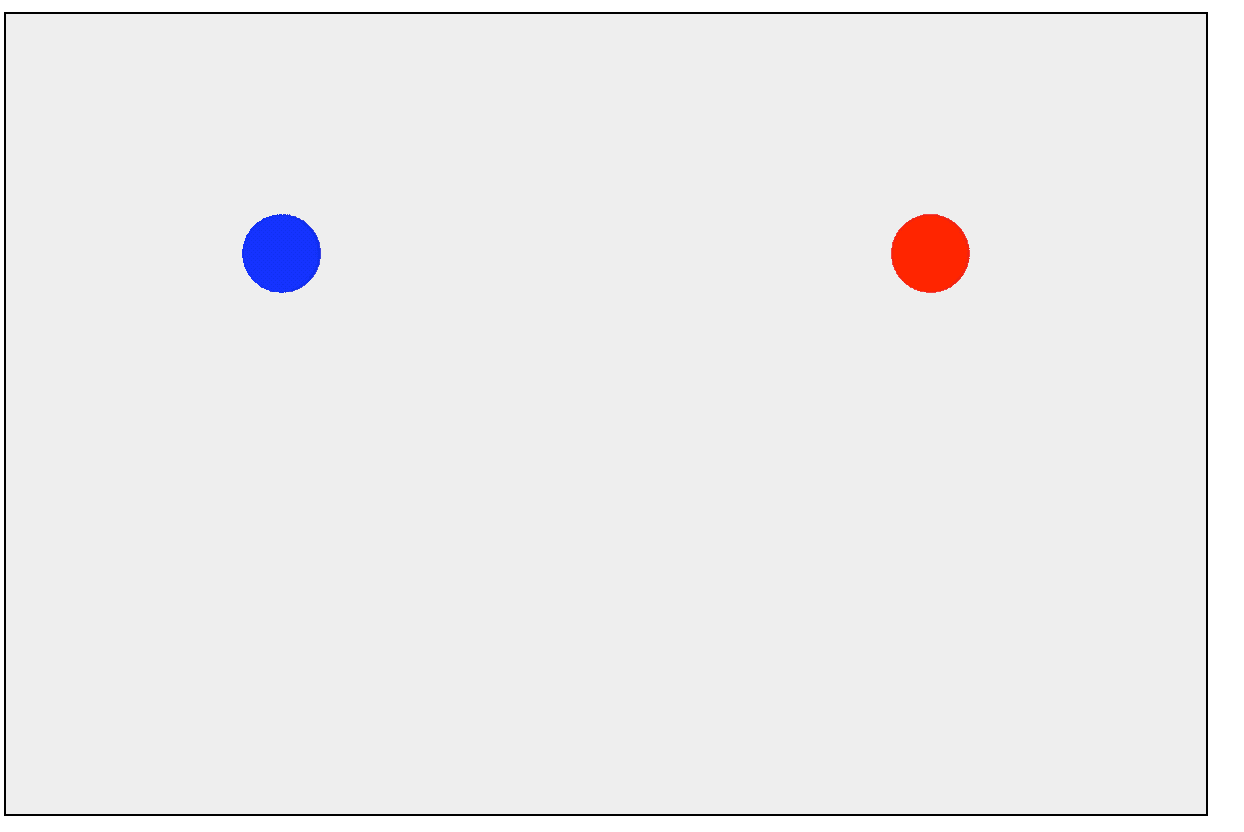## 单轴碰撞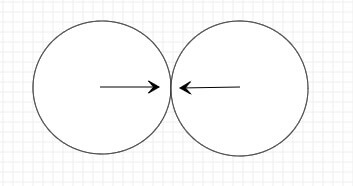1.小球在碰撞前后，系统总动量是相同的；
2.小球在碰撞前后，系统总动能是相同的。

$$m_0 v_0 + m_1 v_1 = m_0 v_{f0} + m_1 v_{f1} ① \\ \frac{1}{2} m_0 v_0 ^ 2 + \frac{1}{2} m_1 v_1 ^ 2 = \frac{1}{2} m_0 v_{f0} ^ 2 + \frac{1}{2} m_1 v_{f1} ^ 2 ②$$

$$由 ① 可得：m_0 ( v_0 - v_{f0} ) = m_1 ( v_{f1} - v_1 ) ③ \\ 由 ② 可得：m_0 ( v_0 ^ 2 - v_{f0} ^ 2 ) = m_1 ( v_{f1} ^ 2 - v_1 ^ 2 ) ④ \\ ④也就是：m_0 ( v_0 - v_{f0} )( v_0 + v_{f0} ) = m_1 ( v_{f1} - v_1 )( v_{f1} + v_1 ) ⑤ \\ 由 ③ 和 ⑤ 可得： v_0 + v_{f0} = v_1 + v_{f1} ⑥ \\ ⑥也就是：v_{f1} = v_0 + v_{f0} - v_1 ⑦ \\ 将 ⑦ 带入 ① 可得：v_{f0} = \frac{ (m_0 - m_1)v_0 + 2 m_1 v_1 }{ m_0 + m_1} \\ 同理可得：v_{f1} = \frac{ (m_1 - m_0)v_1 + 2 m_0 v_0 }{ m_0 + m_1} \\$$

$$V = \frac{4}{3} π R ^ 3 \\ m = ρ V$$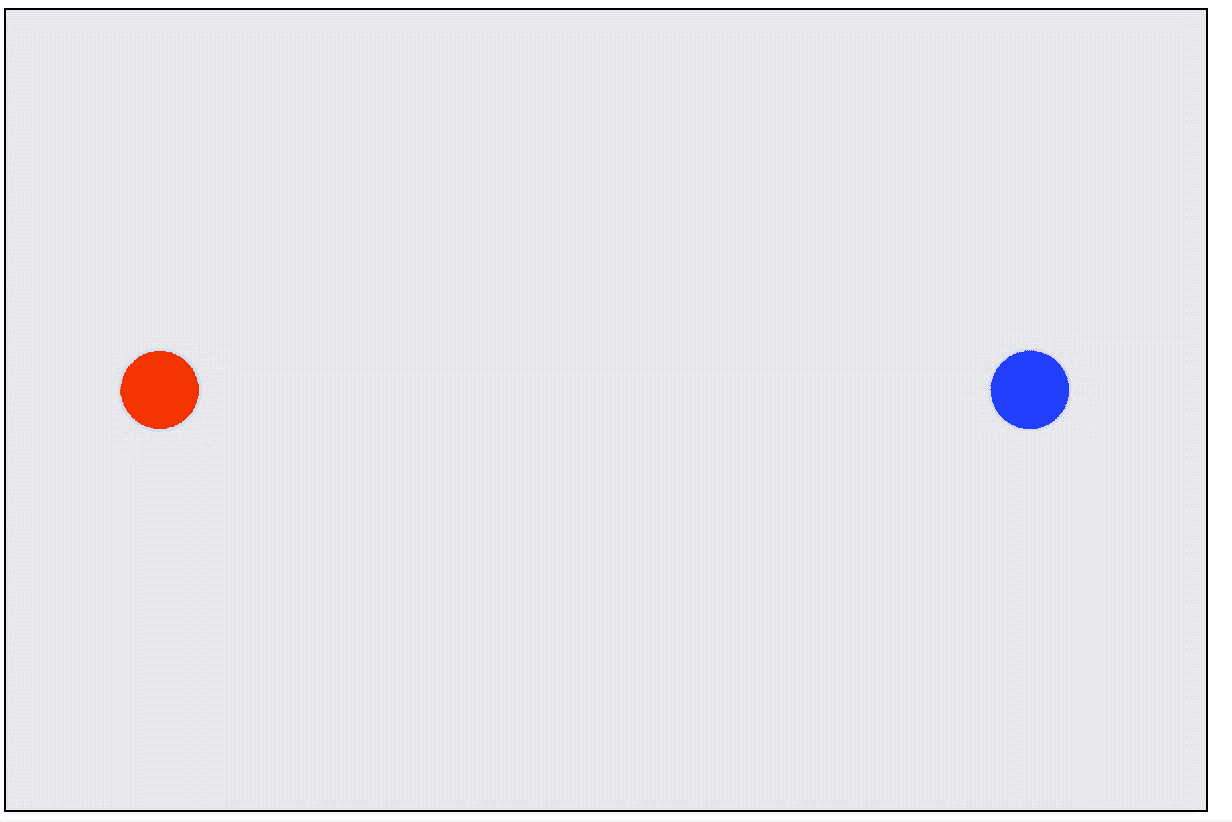## 双轴碰撞理论基础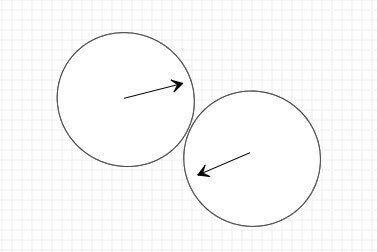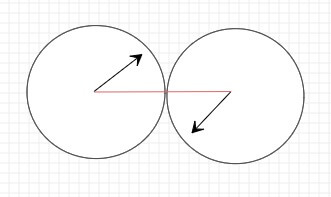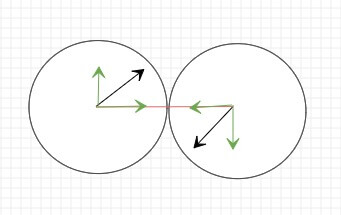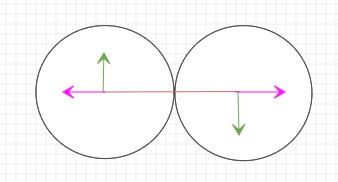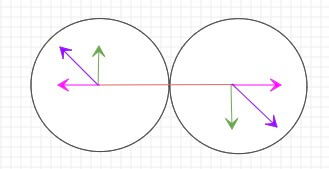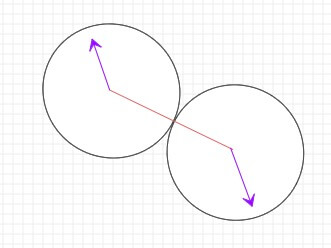## 双轴碰撞的代码实现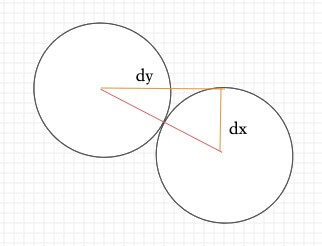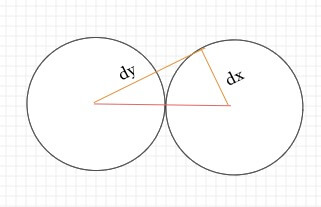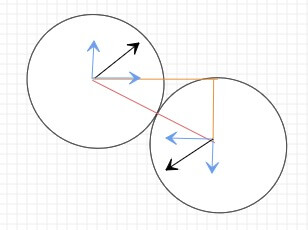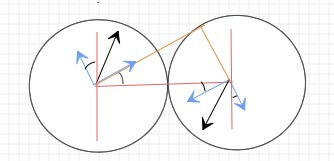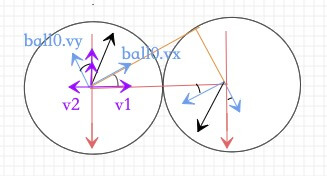$$sin{-\theta} = -sin \theta \\ cos{-\theta} = cos \theta$$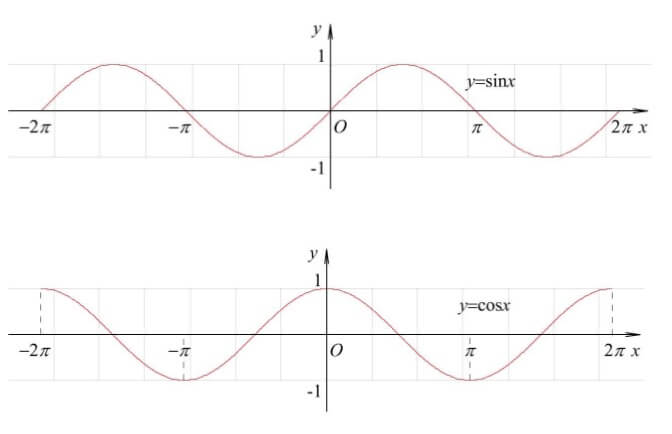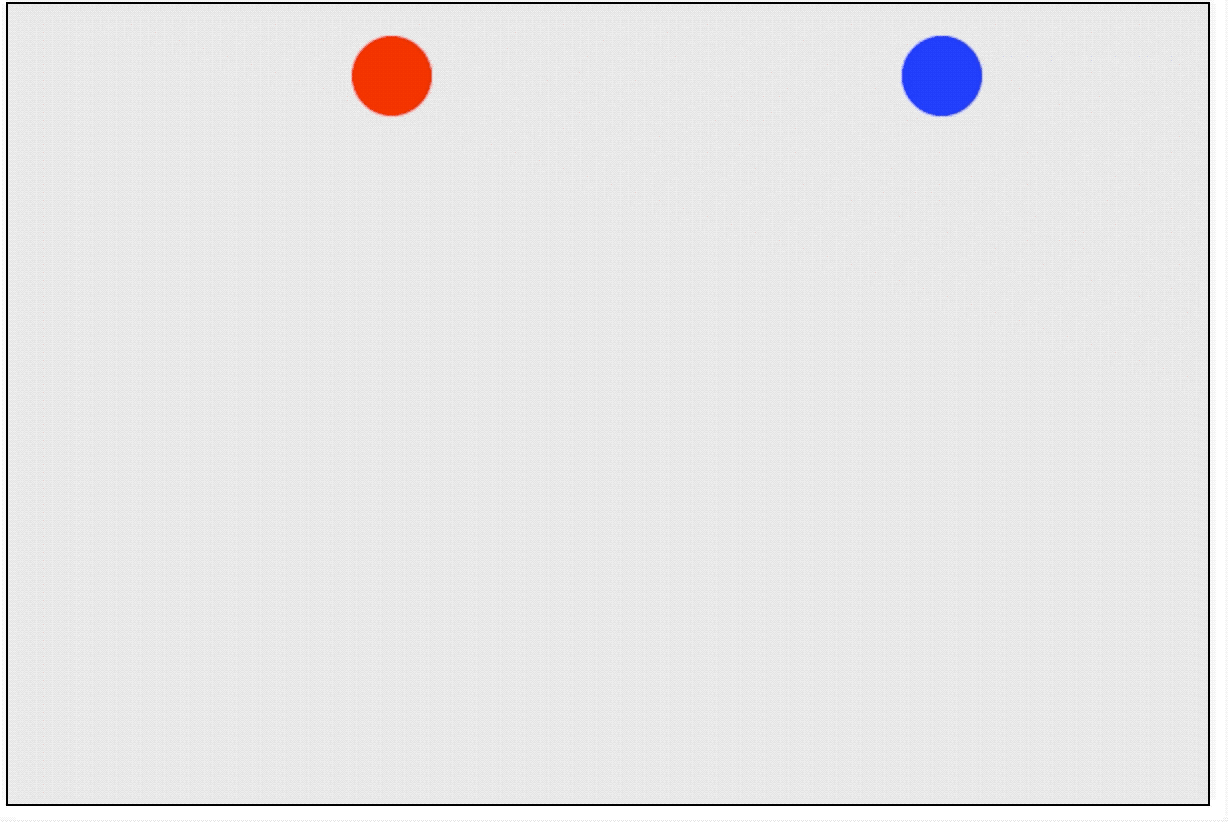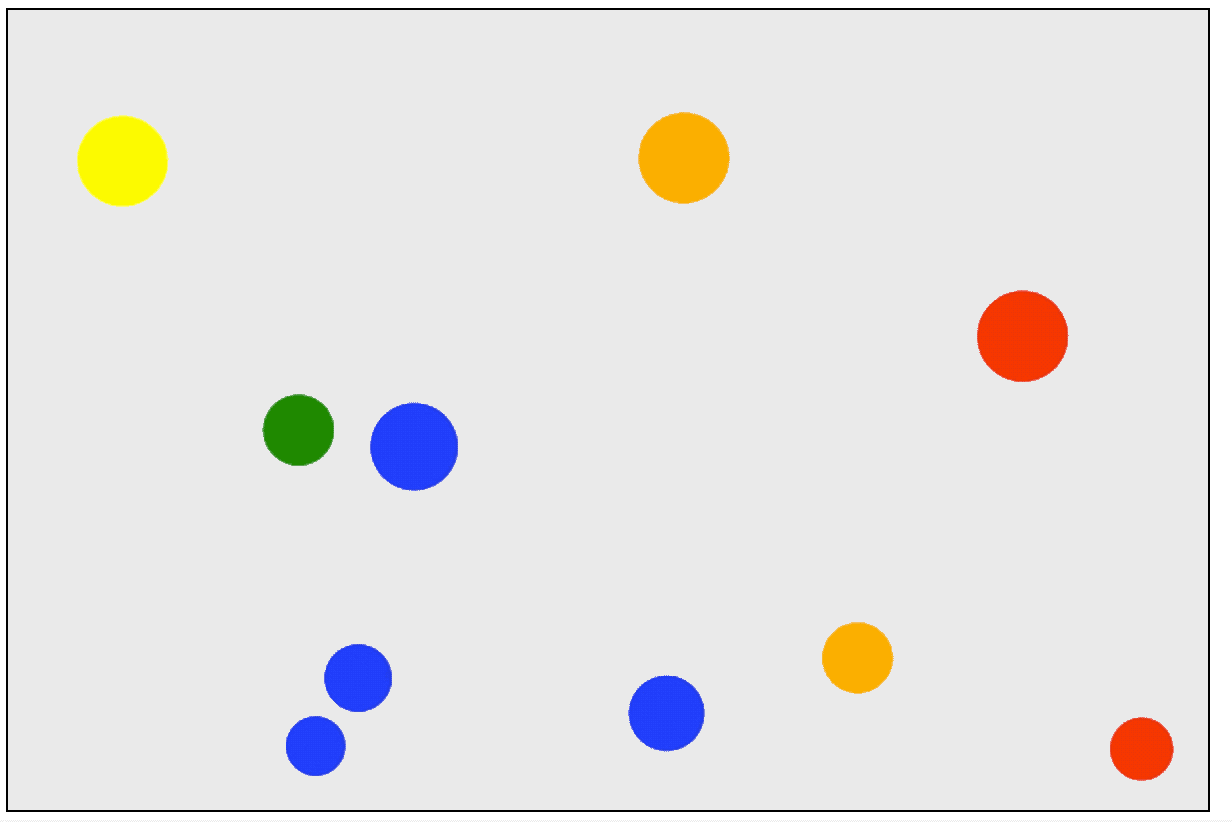-------------本文结束 感谢您的阅读-------------
0%